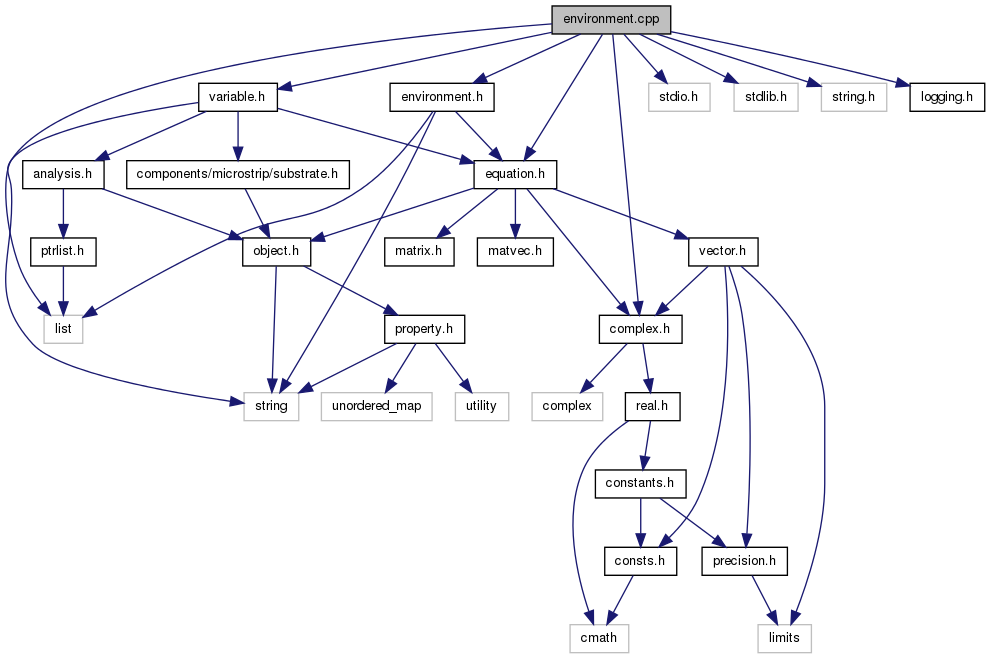Qucs-core  0.0.19
environment.cpp File Reference
`#include <list>`
`#include <stdio.h>`
`#include <stdlib.h>`
`#include <string.h>`
`#include "complex.h"`
`#include "variable.h"`
`#include "equation.h"`
`#include "logging.h"`
`#include "environment.h"`
Include dependency graph for environment.cpp:Go to the source code of this file.

namespace  qucs

## Defines

#define foreach_equation(eqn)
#define A(a)   ((assignment *) (a))

## Define Documentation

 #define A ( a ) ((assignment *) (a))

Definition at line 301 of file environment.cpp.

 #define foreach_equation ( eqn )
Value:
```for (assignment * (eqn) = A (equations);           \
(eqn) != NULL; (eqn) = A ((eqn)->getNext ()))
```

Definition at line 296 of file environment.cpp.